# Histograms and Heatmaps in Python using Plotly

## Histograms and Heatmaps in Python using Plotly

In this article, I am going to discuss Histograms and Heatmaps in Python using Plotly for Data Science with Examples. Please read our previous article where we discussed Box Plots and Dist Plots in Python using Plotly with Examples.

##### Histograms in Python using Plotly

A histogram is a visual depiction of numerical data distribution. Although it resembles a bar graph, a histogram only relates one variable, whereas a bar graph relates two. A histogram necessitates the use of a bin, which divides the full range of values into a series of intervals, and then counts how many values fall into each interval. Bins are often defined as non-overlapping, sequential periods of a variable.

###### For example – let’s create a histogram to check the distribution of tips in tips dataset
```# importing libraries
import plotly.express as px

data = px.data.tips()

# Creating histogram for numerical data
figure = px.histogram(data, x="tip")
figure.show()
```
###### Output: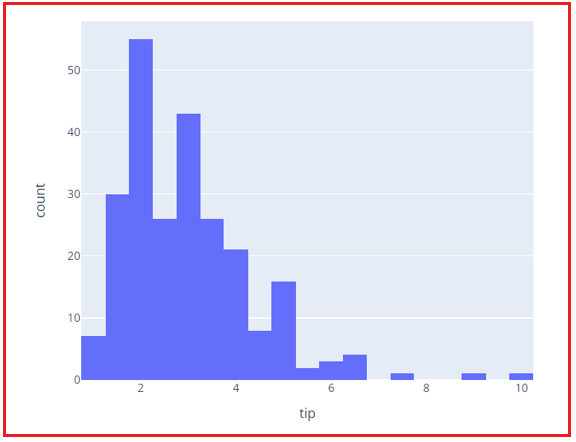###### Example – Let’s check the distribution of day feature
```# importing libraries
import plotly.express as px

data = px.data.tips()

# Creating histogram for categorical data
figure = px.histogram(data, x="day")
figure.show()
```
###### Output: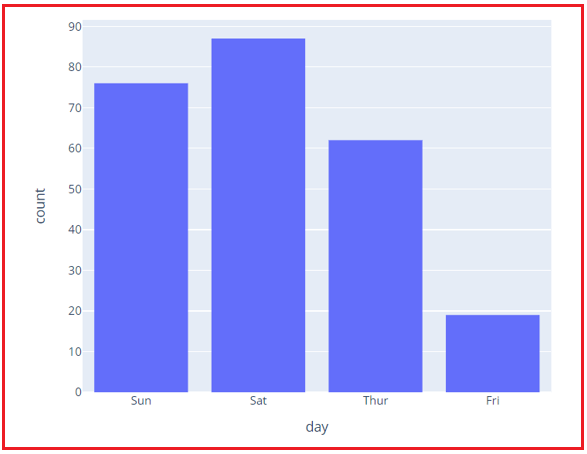###### Example – Let’s see how changing the number of bins can change the distribution visualization
```# importing libraries
import plotly.express as px

data = px.data.tips()

# Creating histogram with defined bins
figure = px.histogram(data, x="tip", nbins=10)
figure.show()
```
###### Output: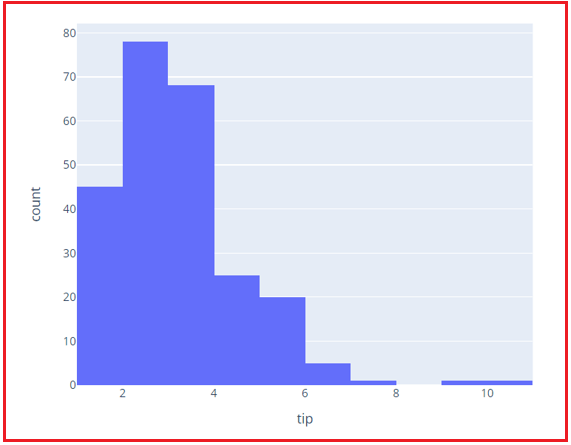###### Example – Let’s check the distribution of tips for a smoker and a non-smoker
```# importing libraries
import plotly.express as px

data = px.data.tips()

# Creating histogram for different values of a feature
figure = px.histogram(data, x="tip", color="smoker", nbins=10)
figure.show()
```
###### Output: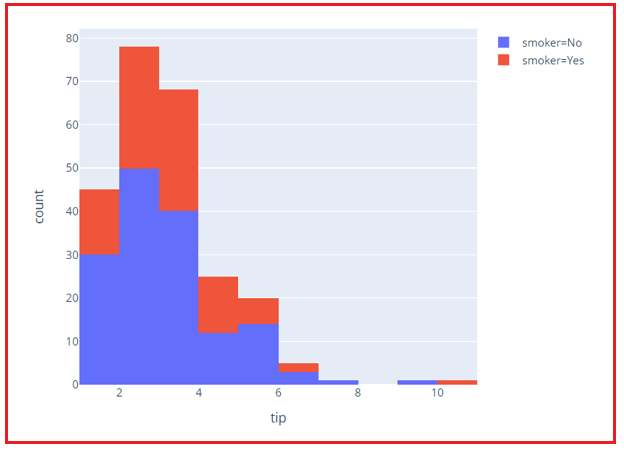##### Heatmaps in Python using Plotly

A heat map (or heatmap) is a graphical representation of data that uses colors to represent the individual values in a matrix. Heat Maps are primarily used to better represent the number of locations/events within a dataset and to guide users to the most important sections on data visualizations. You can create a heatmap by using plotly.express.imshow() function.

###### Example –
```# Import Libraries
import plotly.express as px

# Create an array
arr = [[1,2,3],
[11,12,13],
[21,22,23]]

# Now, let's create a heatmap for this array
figure = px.imshow(arr)
figure.show()
```
###### Output: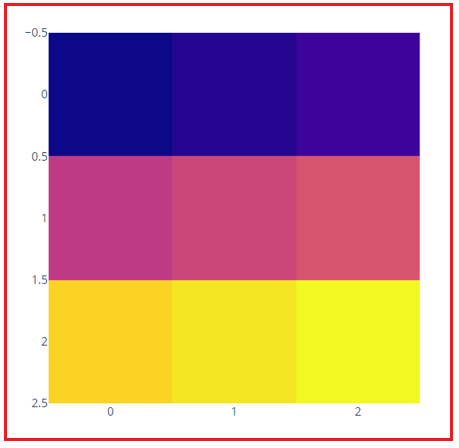Here, in this article, I try to explain Histograms and Heatmaps in Python using Plotly for Data Science with Examples. I hope you enjoy this Histograms and Heatmaps in Python using Plotly for Data Science article.